American Journal of Modern Energy
Volume 2, Issue 5, October 2016, Pages: 22-30

Three-Phase Matrix Converter Based Sliding Mode Controller Applied to Wind Energy Conversion System with Wind Speed Estimation

Alaa Eldien M. M. Hassan, Mahmoud A. Sayed, Essam E. M. Mohamed

Electrical Engineering Department, Faculty of Engineering, South Valley University, Qena, Egypt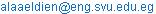(A. E. M. M. Hassan)(M. A. Sayed)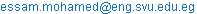(E. E. M. Mohamed)

Alaa Eldien M. M. Hassan, Mahmoud A. Sayed, Essam E. M. Mohamed. Three-Phase Matrix Converter Based Sliding Mode Controller Applied to Wind Energy Conversion System with Wind Speed Estimation. American Journal of Modern Energy. Vol.2, No. 5, 2014, pp. 22-30. doi: 10.11648/j.ajme.20160205.11.

Received: August 17, 2016; Accepted: September 1, 2016; Published: October 28, 2016

Abstract: This paper presents comprehensive modelling ofWind Energy Conversion System (WECS) based on interfacing a Permanent Magnet Synchronous Generator (PMSG) to the utility grid by using the direct AC/AC matrix converter. To estimate the wind velocity and extracts the maximum power at all wind velocities Wind speed estimation control technique is presented based on sliding mode control. Sliding mode controller has many advantages such as fast transient response and robustness against system parametric variations and unknown external disturbances. The matrix converter controls the maximum power point tracking MPPT by adjusting the PMSG terminal frequency, and hence, the shaft speed. In addition, the matrix converter controls the grid injected current to be in-phase with the grid voltage for the unity power factor. Space Vector Modulation is used to generate the PWM signals of the matrix converter switches. The system dynamic performance is investigated using Matlab/Simulink.

Keywords: Permanent Magnet Synchronous Generator (PMSG), Matrix Converter (MC), Sliding Mode Control(SMC)

Contents

1. Introduction

The lack of the non-renerwable sources of energy and environmental pollution form harmful natural disasters. Currently, power generation from renewable energy sources has a great increasing interest and will continue to grow during the coming years. And it will form an intelligent source for electricity generation with minimal environmental impact . Renewable energy sources, such as wind, solar and fossile fule provide clean and cheap energy sources [2-4]. Wind power is now one of the most energy sources that expand rabidly in the industry due to its abundance. Wind Energy is also advantageous over traditional methods of generating energy, in the sense that it is getting cheaper and cheaper to produce energy. Different types of generators are being used with wind turbines such as induction machine, double fed induction machine and PMSG [5-8]. PMSG has become the most familiar type of synchronous generators because of its good features that it has High efficiency and low maintenance cost. In addition, it has Small size and simple construction. There is no need for a separate DC excitation source. On the other hand, PMSG has some drawbacks that it has high initial cost compared with other generators at the same rating. And Overloading, short circuit and high temperate reduce and weaken the magnetization of the permanent magnets [9-11]. The work in this paper is based on PMSG.

The electrical power that generated from the PMSG will vary according to the random variation on the wind velocities, so that this power will not be able to connect to the grid. Therefore, MPPT has been emerged and becoming an essential part in the variable speed wind turbine systems. Many methods have been proposed to locate and track the maximum power point. In [12,13] tip speed ratio (TSR) technique was presented, which depends mainly on measuring the wind velocity, then using the optimal value for the tip speed ratio in order to estimate the optimal value for the shaft speed. In [14-16] power signal feedback (PSF) control technique was presented, which depends upon lookup tables for the shaft speed and its maximum output power at each wind velocity. Also the optimal control technique was presented in some researches [17,18]. The main theory of this method is to adjust the generator torque in order to obtain wind turbine torque for each wind velocity. Perturbation and observation (P&O) method that is independent of the wind velocity detections has been presented in [16,19,20]. This method is based on perturbing the shaft generator speed in small step-size and observing the resulting changes in the output power until the slope becomes zero. The P&O is robust, simple and reliable technique . Wind speed estimation control technique presents in . This method uses the efficiency curve of the blade to estimate the wind velocity without using wind velocity sensor. The work in this paper based on this technique.To control the speed of the PMSG to follow the reference speed that generated from the WSEC controller, Conventional PI-controllers can be applied in the proposed system because of its features as it has designed easily and simple control technique . But, PI-controllers have some drawbacks as its transient response against system parametric variations and unknown external disturbances is slowly [23,24]. Sliding Mode Controller (SMC) has been applied in electric machine drives [25-28]. It is an effective, high frequency switching control strategy for nonlinear systems with uncertainties. It can offer many good features such as high performance against un-modeled dynamics, insensitivity to parameters variation, external disturbance rejection and fast dynamic response .

In order to connect the electrical power to the grid so it converted to DC power then it enter an inverter to convert it to AC power compatible with the grid, this converter called traditional back-to-back converter. but this converter have some drawbacks including: operation on two stages reduces the reliability of the system, the bulky short life-time capacitor on the rectification stage, high power losses, and high amount of harmonics . To overcome the previous mentioned drawbacks of traditional converters the AC/AC matrix converters are applied. Matrix converter is a single-stage AC/AC bi-directional power flow converter that takes power from AC source and converts it to another AC system with different amplitude and frequency. the reduction in the number of switches provide more compact than the traditional converter. Since it has only one power stage, there is no need for the bulky and lifetime limited energy-storing electrolytic capacitor that is considered an essential part in the conventional converters based two stages. It also has the ability to control the output voltage magnitude and frequency in addition to operation at unity power factor for any load. Moreover, it provides sinusoidal input and output waveforms, with minimal higher order harmonics and no sub-harmonics [30,31]. The first attempt for modulation strategy technique of matrix converters is the Venturini method that depends mainly on the product of the input voltage and the modulation index matrix to generate the output voltage. However this method has a poor voltage transfer ratio of 50% . a modification to the strategy proposed in  was presented to enhance the maximum transfer ratio to its maximum limit of 86.7%. The scalar modulation strategy used to utilizes the input voltage to produce the active and non-active states of the converter switches [34-36].

This paper presents a full description of grid-tie wind energy conversion system based on PMSG interfaced to the grid with the direct AC/AC matrix converter. The matrix converter controls the PMSG speed in order to track the maximum power point at all wind speeds based on wind speed estimation control technique. In addition, the matrix converter controls the grid side power factor to be unity in order to inject only active power to the grid. Section I represents the introduction. Section II gives a description of wind turbine and its mathematical model. Section III describes the MPPT control technique based on WSEC method. Section IV gives a full description of the sliding mode controller and its mathematical derivation. Section V matrix converter and its PWM using indirect SVM technique. Section VI presents a control technique of the WECS. The result of this work is presented in section VII. Finally the conclusions are presented in section VIII.

2. Wind Turbine Performance

The wind kinetic energy is converted into mechanical power on the PMSG shaft through the wind turbine. This PMSG converts the mechanical power into electrical power. The mechanical power can be formulatedas follows: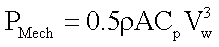(1)

Where: Pmech is the output mechanical power from the turbine in Watts, ρ is the air density in Kg/m3, A is the turbine rotor area in m2 (A=πRr2) and Rr is the rotor blade radius, Cp is the power coefficient and Vw is the wind velocity in m/s. If the air density ρ and the area A are constant, the power coefficient Cp can be formulated as follows:(2)(3)(4)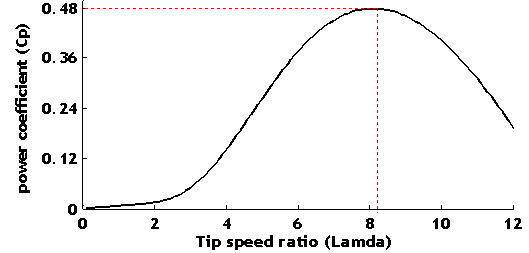Fig. 1. Wind turbine power coefficient versus tip speed ratio.

Where θ is the pitch angle, which is considered zero. Therefore, the tip speed ratio (λ) is formulated as follows:(5)

The optimum value of Cp is about 0.48 for a tip speed ratio of 8.1. In order to keep the system operates at the MPP, the tip speed ratio and power coefficient should be always at this values at all operational conditions , as shown in Fig. (1).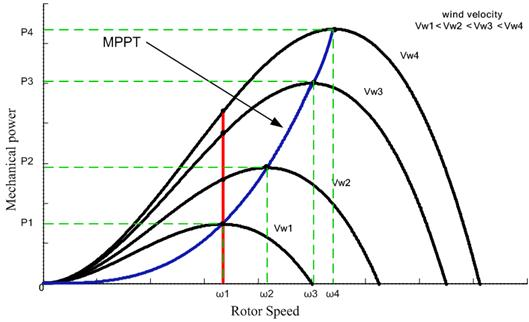Fig. 2. MPPT control scheme at different wind velocities.

3. Maximum Power Point Tracking Control (MPPT)

Fig. 2 shows the relationship between the output mechanical power and shaft speed at different values of wind velocity. According to Fig. 2, for each wind speed there is a unique rotational speed that record maximum mechanical power. Maximum Power Point Tracking (MPPT) is used to extract the maximum mechanical power from the wind turbine at each wind velocity.

It is clear that the maximum power extraction occurs at different rotational speeds. For example, at wind speed (Vw1), the maximum output power is P1, which is obtained at a rotational speed ω1. If the wind speed increases to Vw2, Vw3, or Vw4, applying a constant shaft speed controller to keep the rotational speed constant at ω1 results in a mechanical power less than the maximum available power at the corresponding wind speed. Therefore, in order to extract the maximum power at each wind speed, the rotational speed should be controlled to follow the change in wind speed. If MPPT control is applied, the shaft speed will increase to ω2 at wind speed of Vw2 to extract the maximum power available for this point P2. The same concept applies for the other velocities, i.e. Vw3 and Vw4 .

To estimate the value of the wind velocities at each change the power coefficient (Cp) equations in (2, 3), this nonlinear equation depend on TSR (λ) that formulate in (5). It approximately rewrittenin third order polynomial form to be formulated as in (6):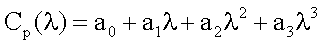(6)

Where: a0 through a3, are constant which can be found numerically. The numerical solution generates values: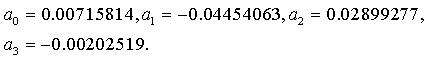Referring to the mechanical power that formulated in (1), after equation (6) substitutethe power coefficient it can be formulated as follow: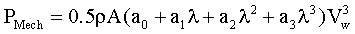(7)

Also equation (5) substitutes the TSR (λ) in (7):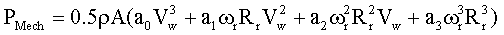(8)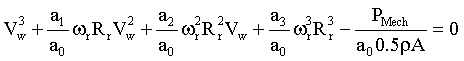(9)

The numerical solution for equation 9 generates three values for the wind velocity. The second answer value is the more accurate empirical solution[38,39].

4. Sliding Mode Controller

MPPT controller is used to track the operating point of the system to achieve maximum values for each change, for the proposed system it used to generate the reference generator speed that achieve the maximum power and force the system to track this speed. PI control can be used in the proposed system to adjust the error signals. Because of the PI control shows a limited performance, and can’t follows the changes in system parameters. This lead to using a nonlinear control in order to accommodate the effects of uncertainties and to provide better robustness over the PI controller.[41,42].

Sliding mode control strategy in the proposed system is used to control the wind turbine speed by regulating the q-axis stator current components (iq) and also will use to regulate the reference d-q axis voltage components (Vd* and Vq*). In order to satisfy the sliding mode condition, define the sliding surface for the speed controller.(10)

ωref is generated by a MPPT method. In addition, in order to determine the stabilizing function, the following Lyapunov function defined as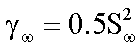(11)

in order to operating the system under the smallest possible error percentage, so that the following condition must be verified: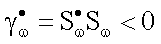(12)

when the sliding mode occurs on the sliding surface, so that: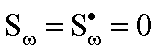(13)

In order to obtain a good dynamic performance for the system, the control must include these two terms: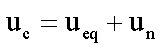(14)

ueq is an equivalent control input that used to control the system’s behavior on the sliding surface. In addition, and also it used to compensate the unknown system dynamics. ueq is calculated from the expression: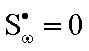(15)

un is used to attract the variable to be controlled towards the sliding surface. It maintains the state on the sliding surface in the presence of the parameter variations and disturbances in the wind variations.(16)

where sgn() is a sign function is defined as:(17)

ϵ is a small positive number. If the is too small or too large, the dynamic quality of the system will be reduced. Applying the derivation for equation (10).(18)

The mechanical equation of PMSG is given by:(19)

Where f is the friction coefficient, J the total moment of inertia and Tm is the mechanical torque produced by wind turbine, Te is electromagnetic torque of PMSG. After substituting the value of ωm from (19).(20)

The electrical torque of the five-phase PMSG can be expressed as: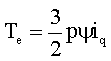(21)

So that (20) can be rewritten as follow(22)

From (13) after appling the sliding mode conditions so that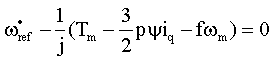(23)

the q- axis current (iq) can be formulated as follows: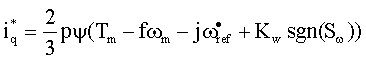(24)

The same mathematic derivation can be performed designed to generate the reference (d-q) axis voltage components from the error of the reference current components (ids, iqs). that can be formulated as follow: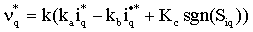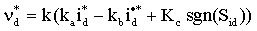(25)

Where k,ka,kb and kc are positive gains depend upon the system parameters.but the value of the d-axis current kept constant (equal zero) so that the derivative of this value also equal zero so the equation of vd* will modify to be.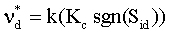(26)

5. Matrix Converter Switching Scheme

Matrix converter is a single stage converter consists of nine bi-directional switches that establish a 3x3 matrix as shown in Fig. 3. In order to avoid short circuit that might be occurred between the input phases and open circuit on the output phases, one and only one switch per column must be ON and the other switches still OFF.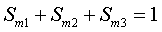where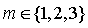(27)

The input three-phase voltage can be formulated as follows:(28)

Where fi is the phase angle of the input voltages waveform.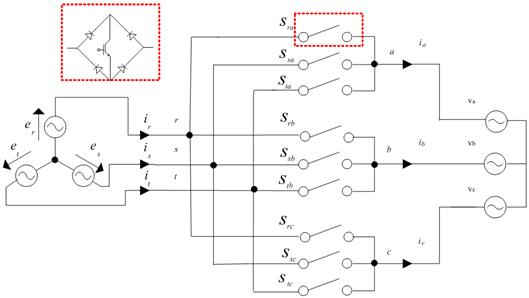Fig. 3. Matrix Converter.

The output three-phase voltage can be formulated as follows as follow:(29)

Where fo is the phase angle of the output voltages waveform.

The relation between the input and the output waveforms can be formulated as follows:(30)

The input current equation is obtained as follows:(31)

Each element in the 3x3 modulation index matrix represents the duty cycle of the switches. These duty cycles of switches are determined by the space vector modulation control.

Space-vector modulation (SVM) is better than conventional PWM techniques because of its advantages as it generates controlled output voltage magnitude and frequency, it generates lower THD and it is suitable for digital controllers . However, the implementation of space vector modulation control technique is complex, and need more switching states.

In order to simplify the states of direct matrix converter, indirect space vector modulation method is considered.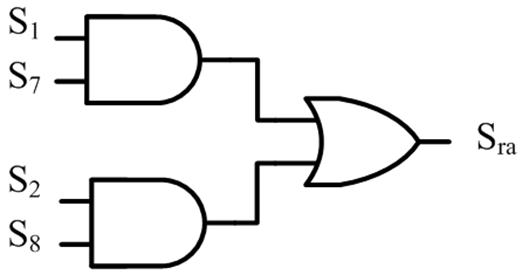Fig. 4. Implementation of the duty cycle of the switch Sra.

The indirect space-vector modulation technique depends on two virtual stages, i.e., the rectification and the inversion stages[45,46]. The modulation index for the matrix that formulated in (30) is subdivided into the product of two matrices, the rectifier matrix and the inverter matrix (32).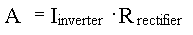(32)

The combination of the rectiﬁer and the inverter duty cycles is used to generate the duty cycles of the nine switches of the Matrix Converter, so that Sra = S1* S7 + S2* S8. This equation is represented using the logic circuit as shown in Fig. 4 . The rectification matrix (S1-S6) represents the duty cycles of the rectification stage switches, and the inversion matrix (S7-S12) represents the duty cycles of the inversion stage switches.

6. Control Scheme of Wind Energy Conversion System

Fig. 5 shows the overall system of the grid-tie wind energy conversion system including PMSG, Matrix converter and its controller circuit, an input filter, and finally the grid side. The wind turbine developed torque Tm is applied to the generator shaft; the wind velocity estimation block is used to estimate the value of the wind velocity which multiplying with the optimum value of the Tip Speed Ratio to generate the referance generator speed that achieves the maximum power extracted at this wind velocity. The reference generator speed is compared with the actual generator speed and the error signal is applied to sliding mode controller to generate the reference value for the q-axis current component. In the other hand the reference value for the d-axis current component (Id*) is set to zero in order to keep the d-axis component flux equals zero. The actual three-phase generator currents are detected and converted to the d-q axis current components (ids-iqs) using Park/Clark Transformation. The actual load current in the d-q axis components are compared with their reference values and the error signal is applied to sliding mode controller to generate the reference d-q axis voltage components Vd* and Vq*. The gains of sliding mode controllers have been tuned depeding on the parameters of the proposed system in order to achieve acceptable transient response. The d-q reference voltage components are converted to the three-phase axis using the invers Park/Clark Transformation in order to obtain the relevant three-phase voltage of matrix converter voltage. Fig.6 Illustrates the block diagram of control scheme of wind energy conversion system.

7. Results

The grid-tie wind turbine model based on PMSG and three-phase-to-three-phase matrix interface converter has been carried out in Matlab/Simulink environment. The parameters of the whole system are listed in Table I. Fig. 7 shows the randomly wind velocity profile that applied in the simulation. Fig. 8 illustrates the estimated and actual wind velocities. Fig. 9 shows the effectiveness of the controller as the feedback speed tracks well the reference speed. Fig. 10 shows the value of Cp that remains constant at its optimal value regardless of the variation in the wind velocities and generator speeds. Fig. 11 shows the Tip Speed Ratio (λ) that remains constant at its optimal value at all values of the wind velocities. The power generated from the machine varies according to the wind velocity variation, as shown in Fig. 12. The generated three-phase currents of the PMSGchange due to the wind speed variation as shown in Fig. 13. Fig. 14 shows the variation in the mechanical and electromagnetic torque on the PMSG shaft. It is clear that both torques are in good agreement. Fig. 15 shows three-phase currents and voltages of the utility grid at all values of wind velocities. fig.16 shows the grid side phase voltage and its corresponding line current at all wind velocites. It is clear that the grid current and voltage are in-phase at all wind speeds. Therefore, the wind turbine injects only active power to the utility grid. Fig.17 and fig.18 provide analysis of the PMSG currents and the gridcurrent waveforms which show that the THD of these currents have a good level of 2% and 9.4% respectively.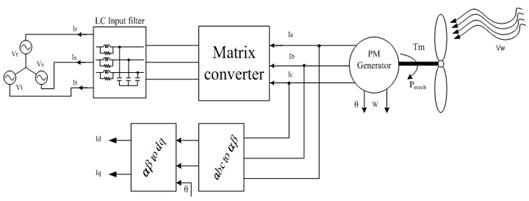Fig. 5. WECS modeling.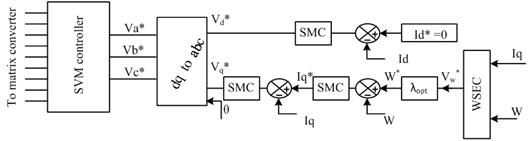Fig. 6. Block diagram of Matrix Converter Controller.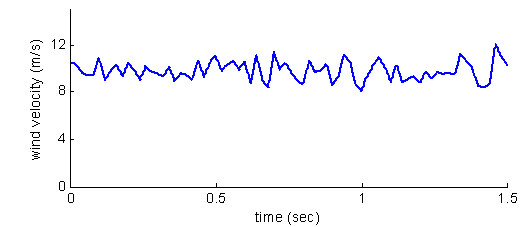Fig. 7. wind velocity profile.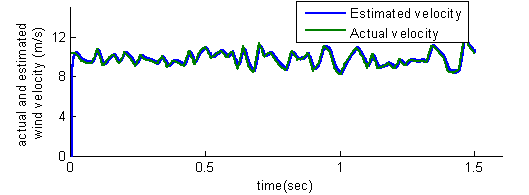Fig. 8. The actual andestimatedwind velocity.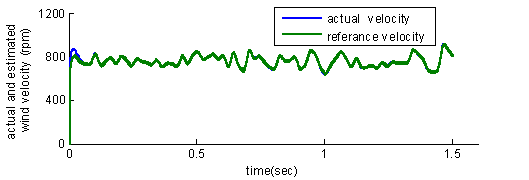Fig. 9. actual and reference generator speed.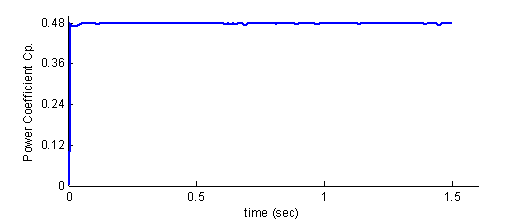Fig. 10. Power Coefficient Cp.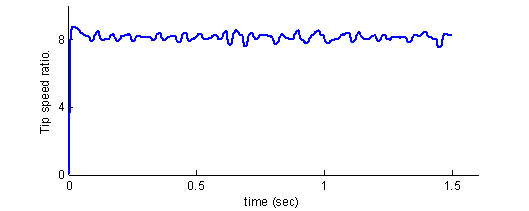Fig. 11. Tip speed ratio.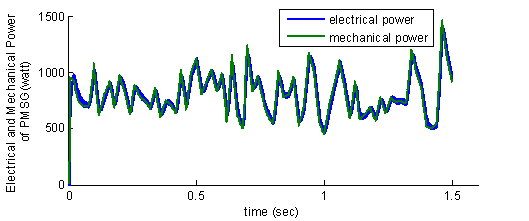Fig. 12. Electrical and Mechanical Power of PMSG.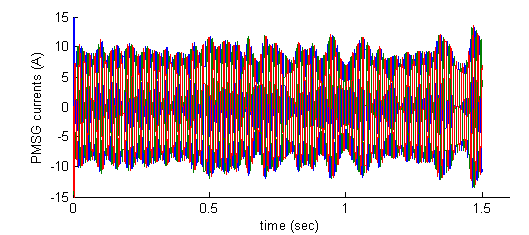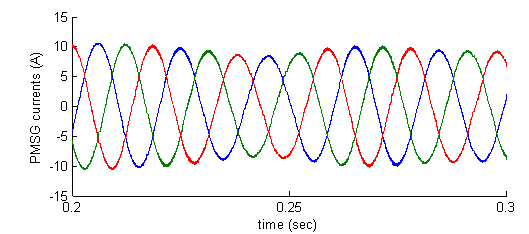Fig. 13. The generator currents variation.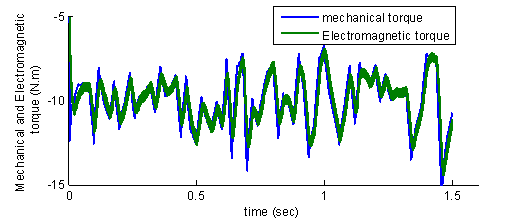Fig. 14.Mechanical and Electromagnetic torque.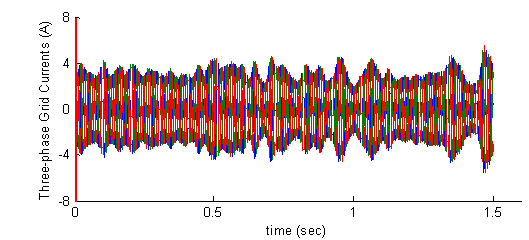Fig. 15. Three-phase Grid Currents.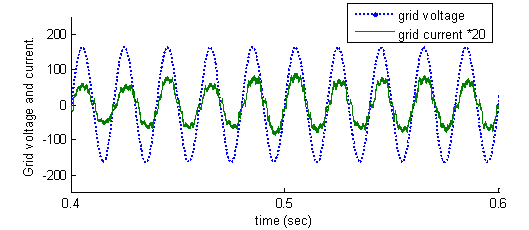Fig. 16.Grid voltage and current.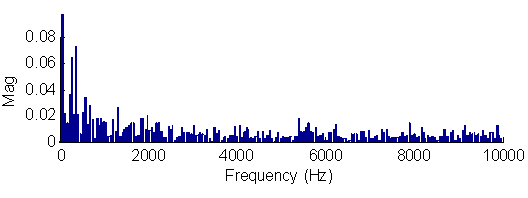Fig. 17. FFT analysis for the Output currents of PMSG.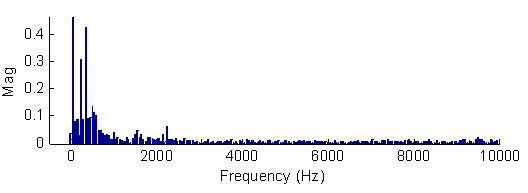Fig. 18. FFT analysis for the grid currents.

8. Conclusions

This paper presents a study of a grid tie WECS for electrical power extraction from wind energy based on PMSG to compensate the shortage of non-renewable energy sources. In order to extract the maximum available power at each wind speed the excellent tracking of the Maximum Power Point based on wind velocity estimation method isapplied. the controller of the MPP based on the sliding mode controller to utlize its benfits over the traditional techniques of speed control. The electrical power generated by the PMG is tied to the grid through a matrix converter, which solve all the problems of the traditional converters. The Matrix Converter, controlled by SVM, enables excellent transient response while sinusoidal current waveforms is dominant with grid currents in-phase with the grid voltage for unity power factor. Simulation results prove that the wind turbine system based three-phase PMSG tracks the MPP and injects only active power to the grid at all wind velocites.

References

1. B. M. Buchholz, Z. Styczynski, and W. Winter, "Dynamic simulation of renewable energy sources and requirements on fault ride through behavior," in Power Engineering Society General Meeting, 2006. IEEE, 2006, p. 7 pp.
2. J. M. Carrasco, L. G. Franquelo, J. T. Bialasiewicz, E. Galván, R. P. Guisado, M. A. Prats, et al., "Power-electronic systems for the grid integration of renewable energy sources: A survey," Industrial Electronics, IEEE Transactions on, vol. 53, pp. 1002-1016, 2006.
3. Z. Olaofe and K. Folly, "Energy storage technologies for small scale wind conversion system," in Power Electronics and Machines in Wind Applications (PEMWA), 2012 IEEE, 2012, pp. 1-5.
4. T. Shanker and R. K. Singh, "Wind energy conversion system: a review," in Engineering and Systems (SCES), 2012 Students Conference on, 2012, pp. 1-6.
5. S. Barakati, M. Kazerani, and X. Chen, "A new wind turbine generation system based on matrix converter," in Power Engineering Society General Meeting, 2005. IEEE, 2005, pp. 2083-2089.
6. V. Agarwal, R. K. Aggarwal, P. Patidar, and C. Patki, "A novel scheme for rapid tracking of maximum power point in wind energy generation systems," Energy Conversion, IEEE Transactions on, vol. 25, pp. 228-236, 2010.
7. Y. Izumi, A. Pratap, K. Uchida, A. Uehara, T. Senjyu, A. Yona, et al., "A control method for maximum power point tracking of a PMSG-based WECS using online parameter identification of wind turbine," in Power Electronics and Drive Systems (PEDS), 2011 IEEE Ninth International Conference on, 2011, pp. 1125-1130.
8. Y. Errami, M. Maaroufi, and M. Ouassaid, "A MPPT vector control of electric network connected Wind Energy Conversion System employing PM Synchronous Generator," in Renewable and Sustainable Energy Conference (IRSEC), 2013 International, 2013, pp. 228-233.
9. T.-F. Chan, "Permanent-magnet machines for distributed power generation: A review," in 2007 IEEE Power Engineering Society General Meeting, 2007, pp. 1-6.
10. H. Polinder, F. F. Van der Pijl, G.-J. De Vilder, and P. J. Tavner, "Comparison of direct-drive and geared generator concepts for wind turbines," Energy conversion, IEEE transactions on, vol. 21, pp. 725-733, 2006.
11. J. Elizondo, M. Macías, and O. Micheloud, "Matrix Converters Applied to Wind Energy Conversion Systems, Technologies and Investigation Trends," in Electronics, Robotics and Automotive Mechanics Conference, 2009. CERMA'09., 2009, pp. 435-439.
12. E. Koutroulis and K. Kalaitzakis, "Design of a maximum power tracking system for wind-energy-conversion applications," Industrial Electronics, IEEE Transactions on, vol. 53, pp. 486-494, 2006.
13. L. Zhang, B. Zhou, F. Cheng, and G. Zuo, "A novel maximum power point tracking control method suitable for a doubly salient electro-magnetic wind power generator system," in World Non-Grid-Connected Wind Power and Energy Conference, 2009. WNWEC 2009, 2009, pp. 1-6.
14. S. M. Barakati, "Modeling and controller design of a wind energy conversion system including a matrix converter," University of Waterloo, 2008.
15. S. M. Barakati, M. Kazerani, and J. D. Aplevich, "Maximum power tracking control for a wind turbine system including a matrix converter," Energy Conversion, IEEE Transactions on, vol. 24, pp. 705-713, 2009.
16. M. Abdullah, A. Yatim, C. Tan, and R. Saidur, "A review of maximum power point tracking algorithms for wind energy systems," Renewable and Sustainable Energy Reviews, vol. 16, pp. 3220-3227, 2012.
17. T. Nakamura, S. Morimoto, M. Sanada, and Y. Takeda, "Optimum control of IPMSG for wind generation system," in Power Conversion Conference, 2002. PCC-Osaka 2002. Proceedings of the, 2002, pp. 1435-1440.
18. S. Morimoto, H. Nakayama, M. Sanada, and Y. Takeda, "Sensorless output maximization control for variable-speed wind generation system using IPMSG," in Industry Applications Conference, 2003. 38th IAS Annual Meeting. Conference Record of the, 2003, pp. 1464-1471.
19. A. Mahdi, W. Tang, and Q. Wu, "Estimation of tip speed ratio using an adaptive perturbation and observation method for wind turbine generator systems," in Renewable Power Generation (RPG 2011), IET Conference on, 2011, pp. 1-6.
20. J. S. Thongam and M. Ouhrouche, "MPPT control methods in wind energy conversion systems," Fundamental and Advanced Topics in Wind Power, pp. 339-360, 2011.
21. H.-S. Shin, C. Xu, J.-M. Lee, J.-D. La, and Y.-S. Kim, "MPPT control technique for a PMSG wind generation system by the estimation of the wind speed," in Electrical Machines and Systems (ICEMS), 2012 15th International Conference on, 2012, pp. 1-6.
22. E. E. Mohamed, M. Sayed, and T. H. Mohamed, "Sliding mode control of linear induction motors using space vector controlled inverter," in Renewable Energy Research and Applications (ICRERA), 2013 International Conference on, 2013, pp. 650-655.
23. A. A. E. Hassan, Y. Sayed, M. T. Hiyama, and T. H. Mohamed, "Model Predictive Control of A Speed Sensorless Linear Induction Motor Drive," in the 14th International Middle East Power Systems Conference, MEPCON’10, December 19-21, 2010.
24. I. Eker, "Sliding mode control with PID sliding surface and experimental application to an electromechanical plant," ISA transactions, vol. 45, pp. 109-118, 2006.
25. V. I. Utkin, "Sliding mode control design principles and applications to electric drives," Industrial Electronics, IEEE Transactions on, vol. 40, pp. 23-36, 1993.
26. W.-J. Wang and J.-Y. Chen, "A new sliding mode position controller with adaptive load torque estimator for an induction motor," Energy Conversion, IEEE Transactions on, vol. 14, pp. 413-418, 1999.
27. M. Rodic and K. Jezernik, "Speed-sensorless sliding-mode torque control of an induction motor," Industrial Electronics, IEEE Transactions on, vol. 49, pp. 87-95, 2002.
28. C.-H. Fang, C.-M. Huang, and S.-K. Lin, "Adaptive sliding-mode torque control of a PM synchronous motor," IEE Proceedings-Electric Power Applications, vol. 149, pp. 228-236, 2002.
29. A. Šabanović and N. Šabanović-Behlilović, "Sliding Modes in Electrical Drives and Motion Control," in The 18th IFAC World Congress, Milano, 2011, pp. 756-761.
30. D. Casadei, G. Grandi, G. Serra, and A. Tani, "Space vector control of matrix converters with unity input power factor and sinusoidal input/output waveforms," in Power Electronics and Applications, 1993., Fifth European Conference on, 1993, pp. 170-175.
31. P. W. Wheeler, J. Rodriguez, J. C. Clare, L. Empringham, and A. Weinstein, "Matrix converters: a technology review," Industrial Electronics, IEEE Transactions on, vol. 49, pp. 276-288, 2002.
32. A. Alesina and M. Venturini, "Solid-state power conversion: A Fourier analysis approach to generalized transformer synthesis," Circuits and Systems, IEEE Transactions on, vol. 28, pp. 319-330, 1981.
33. A. Alesina and M. Venturini, "Analysis and design of optimum-amplitude nine-switch direct AC-AC converters," Power Electronics, IEEE Transactions on, vol. 4, pp. 101-112, 1989.
34. J. Rodriguez, M. Rivera, J. W. Kolar, and P. W. Wheeler, "A review of control and modulation methods for matrix converters," IEEE Transactions on Industrial Electronics, vol. 59, pp. 58-70, 2012.
35. J. Rodriguez, E. Silva*, F. Blaabjerg, P. Wheeler, J. Clare, and J. Pontt, "Matrix converter controlled with the direct transfer function approach: analysis, modelling and simulation," International journal of electronics, vol. 92, pp. 63-85, 2005.
36. L. Zhang, C. Watthanasarn, and W. Shepherd, "Control of AC-AC matrix converters for unbalanced and/or distorted supply voltage," in Power Electronics Specialists Conference, 2001. PESC. 2001 IEEE 32nd Annual, 2001, pp. 1108-1113.
37. M. M. Hussein, M. Orabi, M. E. Ahmed, and M. A. Sayed, "Simple sensorless control technique of permanent magnet synchronous generator wind turbine," in Power and Energy (PECon), 2010 IEEE International Conference on, 2010, pp. 512-517.
38. L. V. Fausett, Applied numerical analysis using MATLAB: Pearson, 2008.
39. W. H. Press, S. A. Teukolsky, W. T. Vetterling, and B. P. Flannery, Numerical recipes in C vol. 2: Citeseer, 1996.
40. S. Muyeen, R. Takahashi, T. Murata, and J. Tamura, "A variable speed wind turbine control strategy to meet wind farm grid code requirements," Power Systems, IEEE Transactions on, vol. 25, pp. 331-340, 2010.
41. F. Valenciaga, P. Puleston, P. Battaiotto, and R. Mantz, "Passivity/sliding mode control of a stand-alone hybrid generation system," IEE Proceedings-Control Theory and Applications, vol. 147, pp. 680-686, 2000.
42. B. Beltran, T. Ahmed-Ali, and M. E. H. Benbouzid, "Sliding mode power control of variable-speed wind energy conversion systems," Energy Conversion, IEEE Transactions on, vol. 23, pp. 551-558, 2008.
43. S. Xue and X. Wen, "Simulation analysis of two novel multiphase SVPWM strategies," in Industrial Technology, 2005. ICIT 2005. IEEE International Conference on, 2005, pp. 1337-1342.
44. M. Y. Lee, P. Wheeler, and C. Klumpner, "Space-vector modulated multilevel matrix converter," Industrial Electronics, IEEE Transactions on, vol. 57, pp. 3385-3394, 2010.
45. M. Jussila and H. Tuusa, "Comparison of direct and indirect matrix converters in induction motor drive," in IEEE Industrial Electronics, IECON 2006-32nd Annual Conference on, 2006, pp. 1621-1626.
46. M. Rivera, J. Rodriguez, B. Wu, J. R. Espinoza, and C. A. Rojas, "Current control for an indirect matrix converter with filter resonance mitigation," Industrial Electronics, IEEE Transactions on, vol. 59, pp. 71-79, 2012.

 Contents 1. 2. 3. 4. 5. 6. 7. 8.
Article ToolsAbstractPDF(780K)